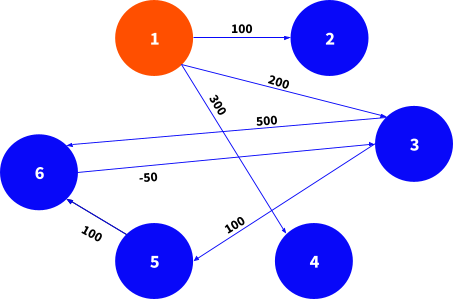November 3, 2022TUTORIAL · MACHINE-LEARNING

# The Bellman-Ford Algorithm

This article explains step-by-step how the Bellman–Ford algorithm may be implemented in Pathway.

## Introduction

The Bellman-Ford algorithm computes the shortest paths from a single source vertex to all the other vertices in a weighted graph. A weighted graph is composed of a set of points, called vertices, which are connected via edges. Each edge is associated to a value, called either weight or distance. For instance, the set of all the cities and the roads which connect them form such a graph. In that example, the Bellman-Ford algorithm would help to find the fastest way, in terms of distance, to go from a given city to another.

## Code

First things first - imports 🙂

``````import math

import pathway as pw``````

### I/O Data

The input is a weighted graph so it is natural to split representation of the data into two parts: Vertices and Edges. Their schemas:

``````class Vertex(pw.Schema):
is_source: bool

class Edge(pw.Schema):
u: pw.Pointer[Vertex]
v: pw.Pointer[Vertex]
dist: int``````

These schemas have a natural interpretation. You can think of the `Edge` schema as of a blueprint of a table that has 3 columns: `u`, `v` (foreign keys) and `dist`. The output schema:

``````class DistFromSource(pw.Schema):
dist_from_source: int``````

Note: The schemas inherit from `pw.Schema` special class.

Note: You might wonder why output schema has only one column `dist_from_source`. Actually, you can join schemas together to create a new one. And so, the output schema is `Vertex + DistFromSource`. (Look for that type annotation later in the code.)

### The algorithm

The Bellman-Ford algorithm performs some number of relaxations until it reaches a fixed point.

#### Relaxations

Each node checks if a path via it would make any so-far-optimal path to some other node shorter.

``````def bellman_ford_step(
vertices_dist: pw.Table[DistFromSource], edges: pw.Table[Edge]
) -> pw.Table[DistFromSource]:
relaxed_edges = edges + edges.select(
dist_from_source=vertices_dist.ix(edges.u).dist_from_source + edges.dist
)
vertices_dist = vertices_dist.update_rows(
relaxed_edges.groupby(id=relaxed_edges.v).reduce(
dist_from_source=pw.reducers.min(relaxed_edges.dist_from_source),
)
)

return vertices_dist``````

#### Fixed point

The relaxations are iterated until a fixed point is reached. In this case, reaching a fixed point means that no new (shorter) path was found in the last iteration.

``````def bellman_ford(vertices: pw.Table[Vertex], edges: pw.Table[Edge]):
vertices_dist: pw.Table[DistFromSource] = vertices.select(
dist_from_source=pw.if_else(vertices.is_source, 0.0, math.inf)
)

fixed_point = pw.iterate(
lambda iterated, edges: dict(
iterated=bellman_ford_step(vertices_dist=iterated, edges=edges)
),
# The `pw.iterate_universe` stanza informs iterate that `vertices_dist` grows with each loop iteration. Without it, the system assumes that iterations don't change the set of indices of a table.
iterated=pw.iterate_universe(vertices_dist),
edges=edges,
).iterated

return fixed_point.join(vertices, fixed_point.id == vertices.id).select(
vertices.key, fixed_point.dist_from_source
)``````

## Tests

Now, let's see the code in action. The following test case runs Bellman-Ford algorithm on a graph depicted below.``````# a directed graph
vertices = pw.debug.table_from_markdown(
"""
| key | is_source
1 | 1   | True
2 | 2   | False
3 | 3   | False
4 | 4   | False
5 | 5   | False
6 | 6   | False
7 | 7   | False
"""
).with_id_from(pw.this.key)

edges = pw.debug.table_from_markdown(
"""
| u  | v | dist
11 | 1  | 2 | 100
12 | 1  | 3 | 200
13 | 1  | 4 | 300
14 | 3  | 5 | 100
15 | 3  | 6 | 500
16 | 5  | 6 | 100
17 | 6  | 3 | -50
"""
).with_columns(
u=vertices.pointer_from(pw.this.u),
v=vertices.pointer_from(pw.this.v),
)``````

Pathway automatically reindexes the tables, so we need a key column of the `vertices` table and we need ask Pathway to reindex the table using those. In practice, Pathway uses pointers so the keys are automatically converted into pointers.

For the edges, we have to convert the keys into their references in order to be able to use `vertices_dist.ix(edges.u)` as `ix` only works with pointers.

``pw.debug.compute_and_print(bellman_ford(vertices, edges))``
``````[2023-12-08T16:29:49]:INFO:Preparing Pathway computation

| key | dist_from_source
^04VMVCM... | 1   | 0.0
^GFBMT58... | 2   | 100.0
^TT1GT7R... | 3   | 200.0
^86W6AGV... | 4   | 300.0
^W788BTV... | 5   | 300.0
^CR88CHY... | 6   | 400.0
^YCS0SGG... | 7   | inf``````

That was a simple introduction to writing code and tests in Pathway.

Feel free to take this code and experiment with it 🙂 Do you see any possibility to improve the code? (What happens when there is a negative cycle in the graph?)

## Summary

The code above follows a pattern that is quite frequent when working with Pathway:

• Define I/O data types
• Define transformations on tables
• Iterate the transformation until a fixed point is reached
• usually transforms the data by a simple one-liner.
• for example `iterate(lambda foo, bar: {foo=fn(foo, bar), bar=bar}, foo=input_table_1, bar=input_table2).foo`

Note: It is also possible to define transformations from the perspective of a row and not of a table. Curious? Take a look at the transformer class tutorial or some of our other cookbooks, like this one on Pagerank.Pathway Team

iteratefixed-pointshortest-pathgraph